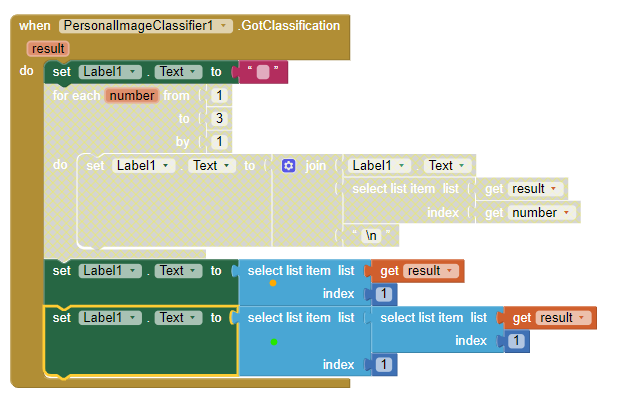# Personal image classifier display

Hi, I'm trying to make an application that recognizes a fruit (within a list of 4) by using a model made with Personal Image Classifier. This model has 4 categories (one for each fruit) but whenever I run the app, it only displays 3 categories. Is there any way to display the 4 categories?

Welcome.

The ImageClassifier only returns the probability percentages for the three highest regardless of the number of 'labels'--classifications. I have 5 classifications; the software will return percentages for the top 3 potential identifications as well as I can determine.

2 Likes

Thank you very much for your explanations!

Hello. Is it possible to display only the HIGHEST probability in the label? If yes, how?

Welcome Areenne.

yes, certainly, the guess and probability in the result is a List. Index #1 is the highest probability.

You canuse the yellow dot Label example to get the object name and its probability
or use the green dot example to post the object name guess
or use the green and change the index from 1 to 2 to post the probability.

Only use one of the Label Blocks.

Is this what you want to do?

1 Like

Yes. Thank you so much Steve. Is it also possible to change the probability to percentage?

Yes, the conversion is simple mathematics. The percentage is the value (for example 0.321 ) multiplied by 100 using the Math Blocks .321 * 100 = 32.1 , use a join to add the word percent (example 32.1 percent probability)

1 Like

Where do I add the math blocks?

Perhaps here

1 Like

Thank you so much!

1 Like

Hello, Steve. I have another question. How do you display the number of qr/barcode scanned by each user?